Trending Today#### GTBank 2019Q3: Time To Boost Loan-Deposit, Drive NPL Ratio DownCompany: GUARANTY TRUST BANK PLC
Current Market Price: N27.00
NPL RATIO (Q3-2019): 5.61%
LOAN to DEBT Ratio: 53.99%
Year High: N39.15
Year Low: N25.70
Fair Value: N33.95
Equity Analyst: Tunde Segun Jeariogbe

Introduction
• This report observed the recently released nine months numbers, representing the performances of Guaranty Trust Bank Plc, compared same with similar figures from the corresponding quarter of 2018 to establish growth and give recommendations accordingly.
• Nevertheless, we have explored numbers from preceding the full-year for projections and valuation purposes.
• We have therefore valued each unit of GTBank shares at N33.95, a marginal drop from our half-year valuation of N36.02 each.
• The result can be considered flat when put side-by-side statistics from the similar period of 2018 statistics, in-fact our Gross Earnings estimates showed a decline from 2018.
• Meanwhile, due to changes in various financial indices that yielded the Profit for the period, the giant financial institution was able to report an improved Profit after Tax for the period, as against corresponding quarter.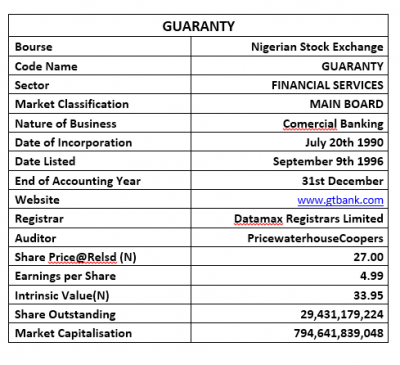• Estimated Gross Earnings for the period stood at N316.38 billion, as against the N317.20 billion in the corresponding period of 2018. The difference between the two estimates is marginal 0.26%.
• In the same manner, Interest Income mildly dropped against that of the corresponding period by 5.62% to the current valuation of N224.18 billion, compared to N237.54 billion.
• Interest Expense dipped by 23.40% at the current N51.25 billion, up from N66.90 billion in 2018.
• Operating Expenses (OPEX) equally adjusted down by 8.29% as it is currently valued at N81.94 billion versus N89.35 billion in the same period of 2018.
• Profit before Tax, therefore, is estimated at N170.65 billion, barely 3.90% above the N164.24 billion of 2018 nine months business session.
• Having provided for Tax Expense, a total of N146.98 billion was reported as Profit for the period, as against N142.22 billion reported in the corresponding period. The percentage difference between the numbers is marginal.
• In the same trend, Total Comprehensive Income grew marginally above that of the corresponding period by 1.81%.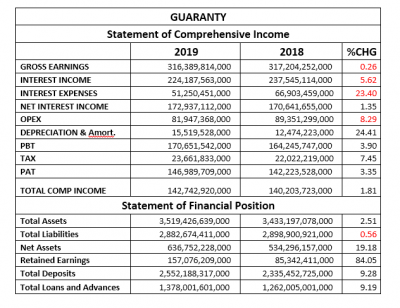• Total Assets of Guaranty Trust Bank is currently valued at N3.519 trillion this is 2.51% improvement over the previous value of N3.433 billion.
• Total Liabilities, on the other hand, inched below previous value. Presently, Total Liabilities is estimated to worth N2.88 trillion versus N2.898 trillion last year.
• Net Assets is now estimated at N636.75 billion against N534.29 billion at the end of the first nine months of 2018.
• Retained Earnings at the end of the quarter is N157.07 billion same as 84.05% above the N85.34 billion achieved at the end of 2018 third quarter.
• Total Deposit improved by marginal 9.28% to stand at the current N2.55 trillion as against N2.33 trillion in the corresponding quarter.
• Similarly, Total Loans & Advances improved slightly by 9.19% against the previous quarter number.

Financial Strength/Solvency Ratios
• We have estimated the Debt Ratio at 0.82x, as against 0.84x last year, in other words, Total Liabilities estimate for the period is the same as 82% of Total Assets valuation. Please note that Total Deposits was added to the Liabilities figure used in the estimate
• We also ascertained that the Total Debt for the period can replicate Equity 4.53x, which is a reduced position from the 5.43x estimated from the 2018 nine-month statistics.
• Total Equity was estimated at 18% of the Asset Value at the end of the period. This is an improvement over the 16% estimated in the corresponding period.
• We have currently estimated a Beta value above the market Beta for Guaranty Trust Bank Plc shares. Note that at 1.56 Beta value, it is well above the 1.34 industry average.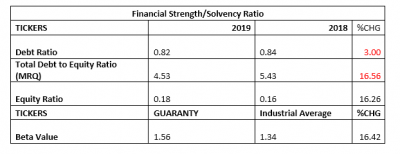Profitability Ratios
• Earnings before Interest, Depreciation, and Amortisation (EBITDA) margin is currently estimated at 67.04%, a 3.67% growth over the previously estimated 64.67% in 2018.
• Pre-Tax Margin stood at 53.94%, up from the last estimate of 51.78% at the end of the 2018 nine months business.
• Interest Expense figure posted for the period is the same as 16.20% of the Estimated Gross Earnings figure; which is a reduced position compared to the previously estimated 21.09%.
• Return on Average Equity used through the period was estimated at 23.08%, lower than the 26.62% return achieved in 2018 numbers.
• See the table below for detailed profitability ratios and comparison.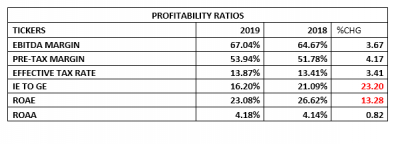Efficiency Ratios
• Operating Expense is estimated at 25.90% of the Gross Earnings Value estimated for the period; this is an improvement in efficiency when compared to the 28.17% estimated from 2018 financial statistics.
• Similarly, Gross Earnings was estimated to be 8.99% of Total Assets, this is a 2.70% marginal drop in efficiency when compared to the estimate from the corresponding period.
• Total Loan to Deposit Ratio achieved at the end of the quarter stood at 53.99%, down from the 54.04% achieved in 2018. Note that this is below the 60% Loan to Deposit Ratio prescribed by the Central Bank of Nigeria (CBN) at the end of the 2019Q3.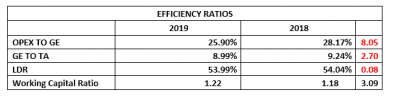Investment/Valuation Ratios
• Earning per unit of Guaranty Trust Bank at the end of the quarter is estimated at N4.99, as against the previously estimated N4.83 each.
• Total Comprehensive Income per unit is estimated for N4.85, versus N4.76 in the corresponding period of 2018.
• Price to Earnings Ratio estimated for the quarter stood at 1.80x, as against 2.53x in 2018.
• The said Earnings Per Share is the same as 18.50% of the current market price of Guaranty Trust Bank Plc on the floor of the Nigerian Stock Exchange (NSE) when the result was released this is a better yield when compared to the previous half-year estimate.
• The current Book Value of Guaranty Trust Bank is N21.64, a 19.18% improvement from the N18.15 estimated last year.
• Confirming a seemingly over-priced position is the Price to Book Value ratio that stood clearly above unity.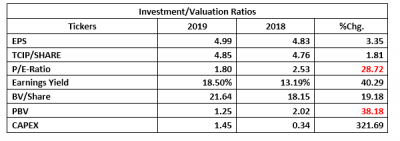Valuation
As noted above, we adjusted down our fair value for each unit of Guaranty Trust Bank Plc to N33.95, from the previous N36.02 each. Considering the defence between the current market price of the equity on the NSE and our achieved fair value, we have rated it a Buy.

## Market Update